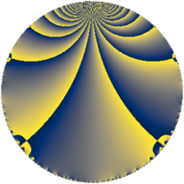Properties

 Label 114.3.gLevel $114$ Weight $3$ Character orbit 114.g Rep. character $\chi_{114}(11,\cdot)$ Character field $\Q(\zeta_{6})$ Dimension $24$ Newform subspaces $1$ Sturm bound $60$ Trace bound $0$

Related objects

Defining parameters

 Level: $$N$$ $$=$$ $$114 = 2 \cdot 3 \cdot 19$$ Weight: $$k$$ $$=$$ $$3$$ Character orbit: $$[\chi]$$ $$=$$ 114.g (of order $$6$$ and degree $$2$$) Character conductor: $$\operatorname{cond}(\chi)$$ $$=$$ $$57$$ Character field: $$\Q(\zeta_{6})$$ Newform subspaces: $$1$$ Sturm bound: $$60$$ Trace bound: $$0$$

Dimensions

The following table gives the dimensions of various subspaces of $$M_{3}(114, [\chi])$$.

Total New Old
Modular forms 88 24 64
Cusp forms 72 24 48
Eisenstein series 16 0 16

Trace form

 $$24 q - 2 q^{3} + 24 q^{4} + 4 q^{6} + 8 q^{7} - 2 q^{9} + O(q^{10})$$ $$24 q - 2 q^{3} + 24 q^{4} + 4 q^{6} + 8 q^{7} - 2 q^{9} - 8 q^{12} + 28 q^{13} - 2 q^{15} - 48 q^{16} + 80 q^{18} - 96 q^{19} + 60 q^{21} - 16 q^{22} - 8 q^{24} - 24 q^{25} - 56 q^{27} + 8 q^{28} + 88 q^{30} + 88 q^{31} + 8 q^{33} - 88 q^{34} + 4 q^{36} - 208 q^{37} - 156 q^{39} + 8 q^{42} + 232 q^{43} - 460 q^{45} - 160 q^{46} - 8 q^{48} + 168 q^{49} - 38 q^{51} - 56 q^{52} + 40 q^{54} + 192 q^{55} - 150 q^{57} + 96 q^{58} + 4 q^{60} - 12 q^{61} + 232 q^{63} - 192 q^{64} - 24 q^{66} + 352 q^{67} + 380 q^{69} + 72 q^{70} + 80 q^{72} - 244 q^{73} + 144 q^{75} + 292 q^{78} + 8 q^{79} - 230 q^{81} - 184 q^{82} + 240 q^{84} + 564 q^{85} + 404 q^{87} - 64 q^{88} + 56 q^{90} - 388 q^{91} - 104 q^{93} - 160 q^{94} - 32 q^{96} - 156 q^{97} + 208 q^{99} + O(q^{100})$$

Decomposition of $$S_{3}^{\mathrm{new}}(114, [\chi])$$ into newform subspaces

Label Dim. $$A$$ Field CM Traces $q$-expansion
$a_{2}$ $a_{3}$ $a_{5}$ $a_{7}$
114.3.g.a $24$ $3.106$ None $$0$$ $$-2$$ $$0$$ $$8$$

Decomposition of $$S_{3}^{\mathrm{old}}(114, [\chi])$$ into lower level spaces

$$S_{3}^{\mathrm{old}}(114, [\chi]) \cong$$ $$S_{3}^{\mathrm{new}}(57, [\chi])$$$$^{\oplus 2}$$